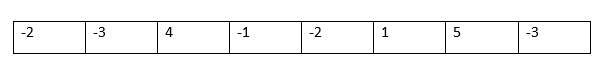# Sub Array with Maximum Sum – Kadane Algorithm

0
1031This is one of the mostly asked question during interviews. Here interviewer loves to see linear algorithmic approach of yours. Yes you can find the maximum sum of elements in linear time using single traversal of the array. Here we will be displaying the sub array. To display sub array with maximum sum you should write code to hold the start and end value of the sub array with maximum sum. Sub Array with Maximum Sum – Kadane Algorithm is the best solution.

Find the sub array with maximum sum

Maximum sum sub array

Sub array with maximum sum

Largest Sum Contiguous Subarray

This can be solved using Kadane’s Algorithm which is a Dynamic Programming approach.

## Find the sum of contiguous subarray in an array of numbers which has the largest sum

You have an array of numbers. Numbers can be positive and negative and in random order. You have to give a set of continuous elements from an array whose sum is maximum. For example, if you have an array of following numbers

Input: -2, -3, 4, 1, -2, 1, 5, -3

Output: 7

#### Method 1

TIME COMPLEXITY:  O(N)
SPACE COMPLEXITY: O(1)

We will use DYNAMIC PROGRAMMING approach. It is also known as Kadane’s algorithm. Also we will print the sub array with maximum sum.```1. Take a sum, maxSum and start variable. Initialize them with Zero
2. Traverse the array using i = 0 till arr.length
3. sum = sum + arr[i]
4. If sum < 0 then sum = 0 and start = i + 1
5. If sum > maxSum then maxSum = sum and end = i
6. Repeat step 3 to 5 till i < arr.length
7. maxSum will be Maximum sum of elements from arr[start] to arr[end]```

## C++

``````#include <bits/stdc++.h>
using namespace std;

int main()
{
int arr[] = {-2, -3, 5, -1, -2, 1, 9, -3,-8,11};

int start = 0; int end = 0;
int maxSum = 0,sum = 0;
int size = sizeof(arr)/sizeof(arr);
for(int i = 0; i < size; i++)
{
sum = sum + arr[i];

if(sum < 0) { sum = 0; start = i + 1; } else if(sum > maxSum) {
maxSum = sum;
end = i;
}
}

cout<<"Maximum sum of the subcontiguous subarray is  " << maxSum<<endl;

cout<<"Elements with Maximum Sum are" <<endl;

for(int i = start; i <= end; i++)
{
cout<<arr[i]<<" ";
}

return 0;
}``````

## Java

``````
import java.util.Arrays;

public class Main {

public static void main(String[] args) {

int arr[] = {-2, -3, 5, -1, -2, 1, 9, -3,-8,11};

int start = 0; int end = 0;
int maxSum = 0,sum = 0;
int i;
for(i = 0; i < arr.length; i++)
{
sum = sum + arr[i];

if(sum < 0) { sum = 0; start = i + 1; } else if(sum > maxSum) {
maxSum = sum;
end = i;
}
}

System.out.print("Elements with Max Sum are ");
for(i = start; i <= end; i++)
System.out.print(arr[i] + " ");
System.out.println(" and Max Sum is " + maxSum);

}
}
``````

#### Method 2

TIME COMPLEXITY: O(N2)
SPACE COMPLEXITY: O(N)

Simply use Brute Force way by obtaining all the subarray sums and find the maximum among them.

1.    Take an element
2.    Loop from the next element to the end by computing sum at each step and
3.    Compare the sum at each step with the overall maximum

At the end when we compute all the N*(N+1)/2 subarrays we get the maximum.

``````#include <bits/stdc++.h>
using namespace std;

int main()
{
int arr[] = {-2, -3, 4, -1, -2, 1, 5, -3};
int size = sizeof(arr)/sizeof(arr);

int max_sum = INT_MIN;
for(int i=0;i<size;i++)
{
int temp_sum = 0;
//find all the subarrays and their sum
for(int j=i;j<size;j++)
{
temp_sum += arr[j];

//compare maximum of these subarrays with the overall max obtained till now
if(temp_sum > max_sum)
max_sum = temp_sum;
}
}
cout<<"Maximum sum of the subcontiguous subarray is  " << max_sum<<endl;

return 0;
}``````

#### Conclusion

Sub array with maximum sum is one of the mostly asked questions in product based companies during technical interviews. Try to practice and explain the logic of Kadane’s algorithm when they ask you to explain.

#### References

Maximum Subarray Problem